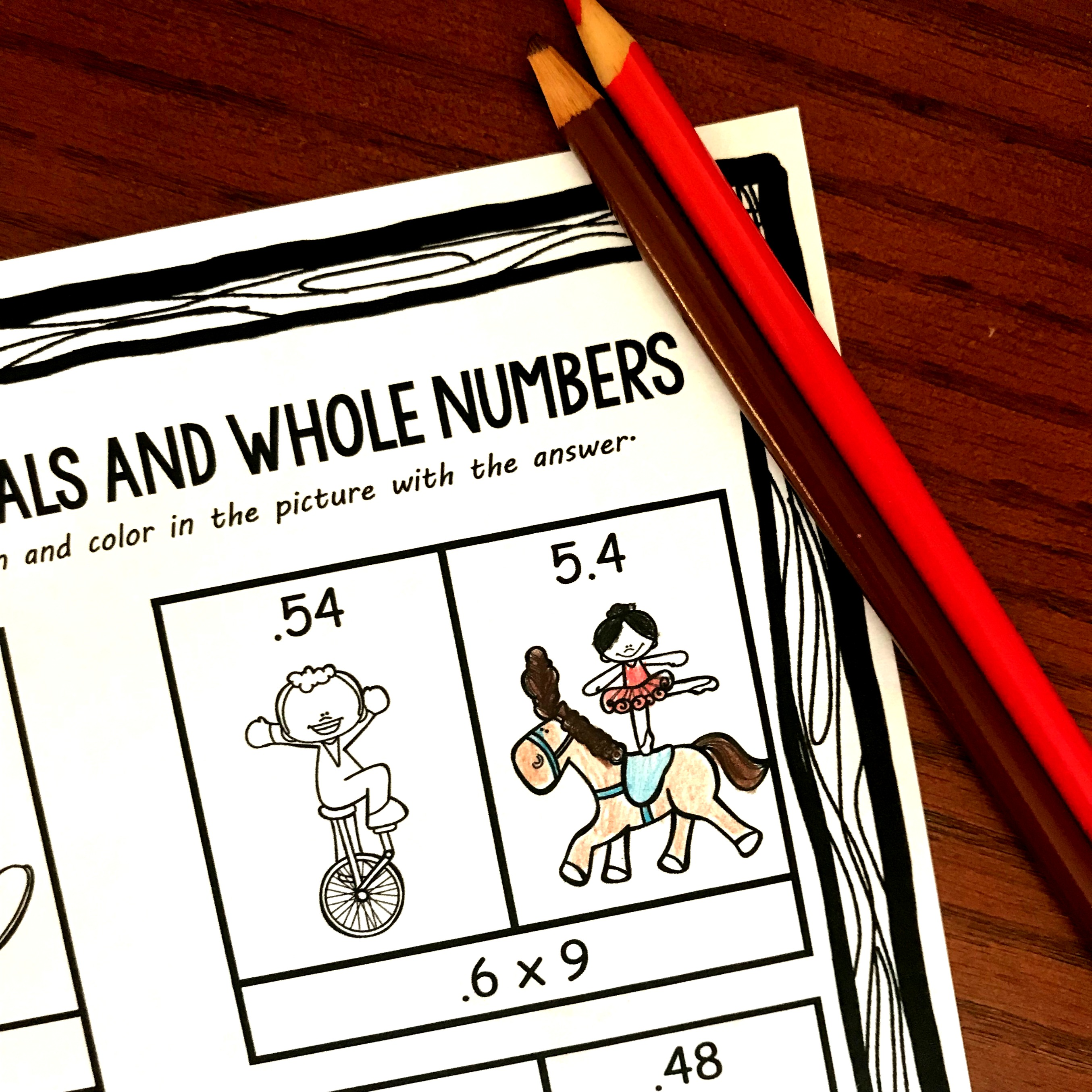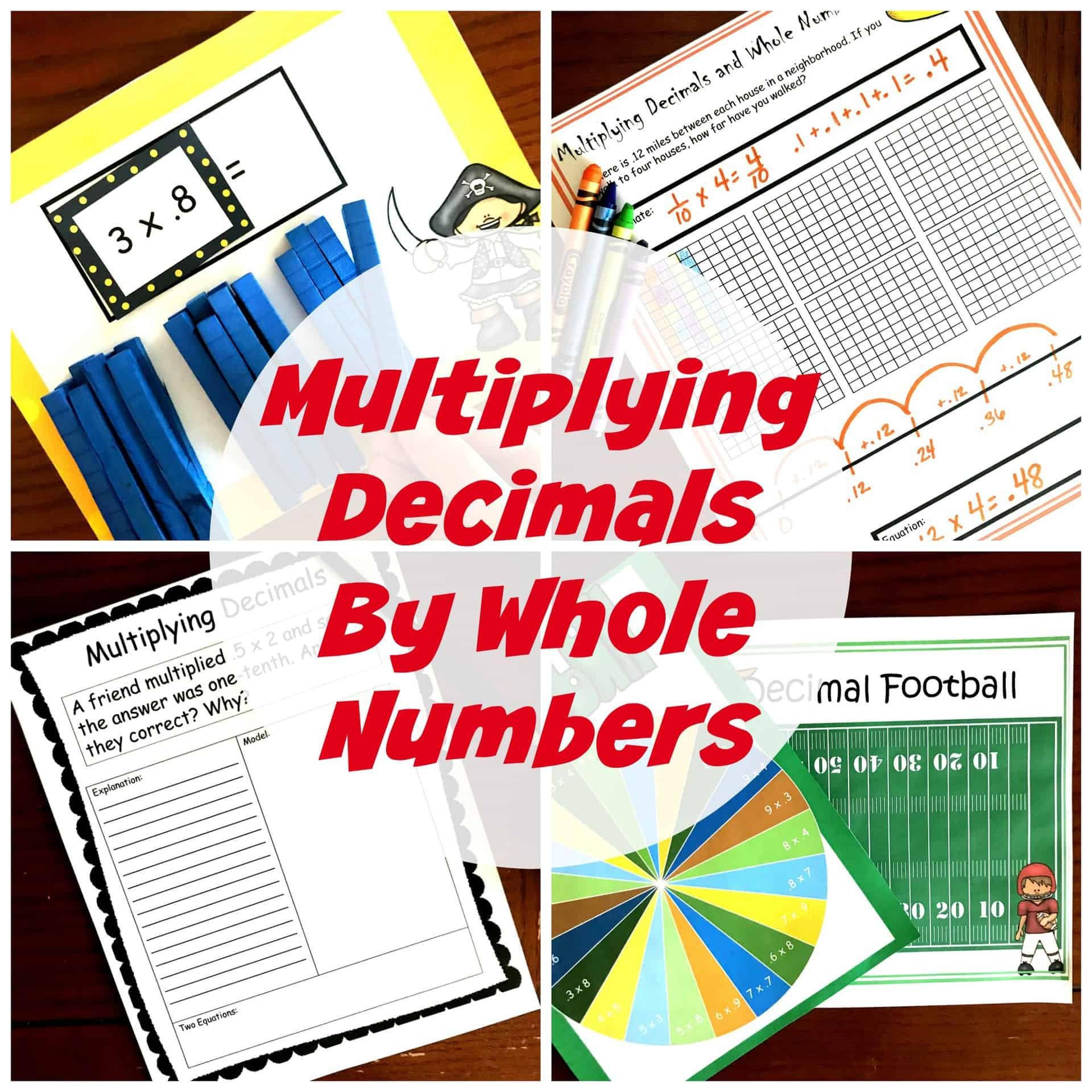Home » Math » FREE Multiplying Decimals and Whole Numbers Coloring Worksheet

# FREE Multiplying Decimals and Whole Numbers Coloring Worksheet

These free pages have children Multiplying Decimals and Whole Numbers (.2 x 9) and then coloring in the correct answer. Focuses on where the decimal should go.Do you know what the hardest part of multiplying decimals is? Knowing where to put the decimals.

Other than knowing where that silly decimal goes, it is just like multiplying numbers.

SO today, I have some very simple coloring pages that has children multiplying decimals and whole numbers and coloring in the answer that has the decimal in the correct place.

## Prep-Work

No need to stress with this one. It is a print and go… type activity.

1. First, print off the pages you want to use.
2. Next, provide crayons and base ten blocks.
3. And you are ready to go.

## Using Base Ten Blocks For Multiplying Decimals and Whole Numbers

If you have read any of my blog, you know I’m very passionate about not just teach our children a formula but letting them see WHY it works. I’m not saying that every time they do a decimal problem they pull out their base ten blocks. What I am saying is that at the beginning, children should be able to draw, design, and manipulate objects to SEE why the formula works. And after that foundation has been laid, then we move on to just having them do the formula.

There are very few problems on these sheets, so it is a good one to ask the children to show their work.

If you are not sure how base ten blocks work with decimals, you can start with these task cards. The complete decimal grid is a flat, each column is a long, and the small squares equal the cubes.Here’s how it goes.

I need to multiply .6 x 9.

I begin by making nine circles on my paper. This is how many groups I will have. Then I put six tenths (6 longs) in each circle.

I ended up with 54 longs. I can’t write 54 in the tenths place, so it is time for some regrouping.

Every 10 longs equals one whole.

So I end up with 5 flats which equals 50 longs.

There are four longs left over. This means I have five wholes and four tenths, and we write it like this. 5.4

By working out the problem with base ten blocks our children don’t just learn the rule count the decimals and move over that many spaces, the begin to understand why it works.

I hope they enjoy this easy activity as they figure out where the decimal goes when multiplying decimals and whole numbers.

Need more hands-on multiplying decimal work? This may help!!!You’ve Got This,

Rachel